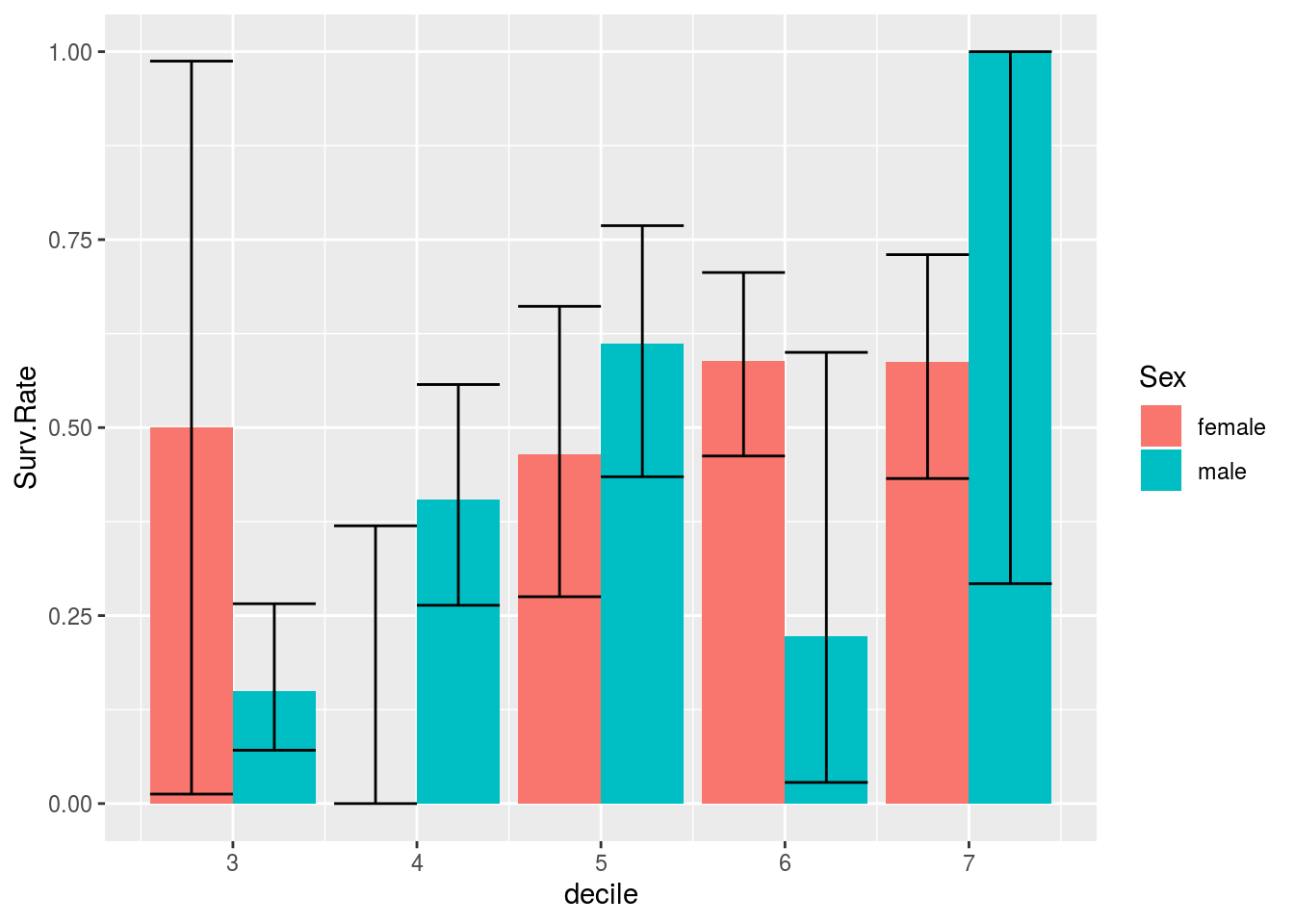### Problem 3

titanic <- read.csv("http://guerzhoy.princeton.edu/201s20/titanic.csv")

Learning goals

• Understand calibration and FPR parity
• Be able to implement checks for calibration and quantities such as FPR/PPV/etc. parity

#### False Positive Parity

get.FPR <- function(fit, data){
dat.neg <- data %>% filter(Survived == 0)
pred <- predict(fit, newdata = dat.neg, type = "response") > 0.5
mean(pred == 1)
}

fit <- glm(Survived ~ Sex + Age + Pclass, family = binomial, data = titanic)
FPR.m <- get.FPR(fit, titanic %>% filter(Sex == "male"))
FPR.f <- get.FPR(fit, titanic %>% filter(Sex == "female"))
data.frame(FPR.m = FPR.m, FPR.f = FPR.f)
##        FPR.m     FPR.f
## 1 0.03448276 0.8148148

The false positive rates vary quite dramatically between female and male passengers. There is no false positive parity.

#### Calibration

get.PPV <- function(fit, data){
data$pred <- predict(fit, newdata = data, type = "response") > 0.5 data.predpos <- data %>% filter(pred == 1) mean(data.predpos$Survived == 1)
}

get.NPV <- function(fit, data){
data$pred <- predict(fit, newdata = data, type = "response") > 0.5 data.predneg <- data %>% filter(pred == 0) mean(data.predneg$Survived == 0)
}

fit <- glm(Survived ~ Sex + Age + Pclass, family = binomial, data = titanic)
PPV.m <- get.PPV(fit, titanic %>% filter(Sex == "male"))
PPV.f <- get.PPV(fit, titanic %>% filter(Sex == "female"))
NPV.m <- get.NPV(fit, titanic %>% filter(Sex == "male"))
NPV.f <- get.NPV(fit, titanic %>% filter(Sex == "female"))
data.frame(sex = c("m", "f"), PPV = c(PPV.m, PPV.f), NPV = c(NPV.m, NPV.f))
##   sex       PPV      NPV
## 1   m 0.4666667 0.825046
## 2   f 0.7755102 0.750000

Calibration is also not satisfied.

#### Extra material

We can try to see whether calibration is approximately satisfied by looking at the “decile scores” that we can obtain for the Titanic data:

fit <- glm(Survived ~ Sex + Age + Pclass, family = binomial, data = titanic)
titanic$decile <- round(predict(fit, newdata = titanic, type = "response")*10) titanic.surv <- titanic %>% group_by(decile, Sex) %>% summarize(Surv.Rate = mean(Survived), Surv.Total = sum(Survived), n = n()) %>% rowwise() %>% mutate(surv.min = binom.test(Surv.Total, n, p = Surv.Rate)$conf.int,
surv.max = binom.test(Surv.Total, n, p = Surv.Rate)$conf.int) %>% ungroup() %>% group_by(decile) %>% filter(n() > 1) ggplot(titanic.surv, mapping = aes(x = decile, y = Surv.Rate, fill = Sex)) + geom_bar(stat = "identity", position = "dodge") + geom_errorbar(mapping = aes(ymin = surv.min, ymax = surv.max), position = "dodge") + scale_x_continuous(breaks = c(0:10))We’d expect equal survival probabilities for the same deciles if there is calibration. As you can see, that is not exactly the case; furthermore, there are no male passangers at all in the top three deciles. We can try again, without including sex as a predictor: fit <- glm(Survived ~ Sex + Age + Pclass, family = binomial, data = titanic) titanic$decile <- round(predict(fit, newdata = titanic, type = "response")*10)

titanic.surv <- titanic %>% group_by(decile, Sex) %>%
summarize(Surv.Rate = mean(Survived), Surv.Total = sum(Survived), n = n()) %>%
rowwise() %>%
mutate(surv.min = binom.test(Surv.Total, n, p = Surv.Rate)$conf.int, surv.max = binom.test(Surv.Total, n, p = Surv.Rate)$conf.int) %>%
ungroup() %>%
group_by(decile) %>%
filter(n() > 1)

ggplot(titanic.surv, mapping = aes(x = decile, y = Surv.Rate, fill = Sex)) +
geom_bar(stat = "identity", position = "dodge") +
geom_errorbar(mapping = aes(ymin = surv.min, ymax = surv.max), position = "dodge") +
scale_x_continuous(breaks = c(0:10))We can’t reject the idea that there is calibration here as well. But since sex is a predictor of survival, we’d expect that there would be no calibration.